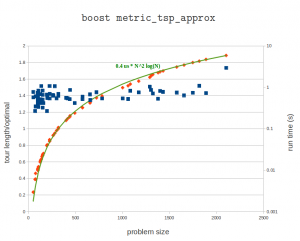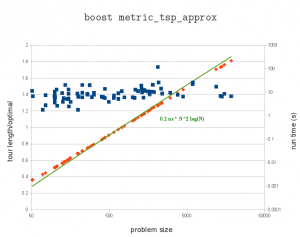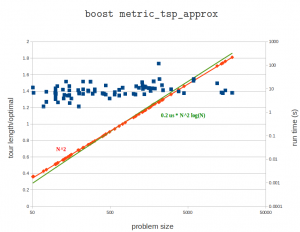## TSP

If you have lots and lots of holes to drill on your cnc-machine you might want a TSP solver to optimize the order of the holes so as to minimize rapid movements between holes. I've written a small wrapper around metric_tsp_approx from the boost graph library and included it in opencamlib as a new class TSPSolver. Here's an example on problem "u2152" from TSPLIB95.Here's a plot of the tour length compared to the known optimal tour length, as well as the run-time on my laptop (P9400 CPU). These problems range in size from 51 points to 2103 points. I might run the bigger problems on the desktop machine later. The algorithm guarantees a tour which is at worst twice the length of the optimal tour. In practice the solutions seem to scatter around 1.4 or 1.5 times the length of the optimal tour. The run times fall neatly on the predicted O(N²*log(N) curve.

A simple greedy heuristic (with better run-time?) which always selects the closest unvisited point also guarantees a tour at most twice the length of the optimal tour.

There's also a better heuristic, the Christofides algorithm, which guarantees a tour length at most 1.5 times the optimal tour length. In addition to the minimum-spanning-tree algorithm it requires a minimum cost perfect matching algorithm. I'm not sure if there is open-source code for this somewhere.Update: here's a run on an i7-2600K cpu. The pre-factor is now halved to about 0.2 microseconds. The largest problem has 18512 cities and takes over three minutes (217 seconds) to solve.Here's 'usa13509' from TSPLIB. Cities with population at least 500 in the continental US. Presumably from 1995 or so.Scaling (?)## Matrix determinant with Boost::uBLAS

Boost uBLAS provides BLAS functionality, but doesn't have a function for computing the determinant of a matrix. Googling for this turns up a few code snippets, but it's best to document this completely here now since I got it to work, and it will be useful for opencamlib sooner or later.

```1 2 3 4 5 6 7 8 9 10 11 12 13 14 15 16 17 18 19 20 21 22 23 24 25 26 27 28 29 30 31 32 33 34 35 36 37 38 39 40 41 42 #include <boost /numeric/ublas/matrix.hpp> #include </boost><boost /numeric/ublas/io.hpp> #include </boost><boost /numeric/ublas/lu.hpp>   namespace bnu = boost::numeric::ublas;   int determinant_sign(const bnu::permutation_matrix<std ::size_t>& pm) { int pm_sign=1; std::size_t size = pm.size(); for (std::size_t i = 0; i < size; ++i) if (i != pm(i)) pm_sign *= -1.0; // swap_rows would swap a pair of rows here, so we change sign return pm_sign; }   double determinant( bnu::matrix<double>& m ) { bnu::permutation_matrix</std><std ::size_t> pm(m.size1()); double det = 1.0; if( bnu::lu_factorize(m,pm) ) { det = 0.0; } else { for(int i = 0; i < m.size1(); i++) det *= m(i,i); // multiply by elements on diagonal det = det * determinant_sign( pm ); } return det; }   int main () { bnu::matrix<double> m(3, 3); for (unsigned i = 0; i < m.size1() ; ++i) { for (unsigned j = 0; j < m.size2() ; ++j) { m (i, j) = 3 * i + sqrt(j+1); // fill matrix m(i,j) = m(i,j)*m(i,j); // with some numbers } } std::cout << "before det() call m= " << m << std::endl; double det = determinant(m); std::cout << "after det() call m= " << m << std::endl; // m has changed afted determinant() call! std::cout << "determinant=" << det << std::endl; }```

I'm trying the WP-syntax plugin here for the first time. The include statements are garbled, but otherwise it seems to work.

```cmake_minimum_required(VERSION 2.6)